# HSPT Math : Arithmetic

## Example Questions

### Example Question #21 : Concepts

Evaluate: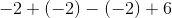Explanation:

Rewrite the expression by simplifying the signs.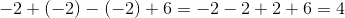### Example Question #21 : Arithmetic

Solve: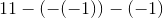Explanation:

Simplify the negative signs to a single sign and solve.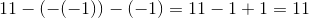### Example Question #22 : Arithmetic

Solve: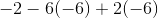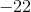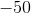Explanation:

To evaluate, simplify the parenthesis first.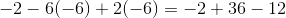Combine the terms.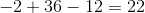### Example Question #22 : Concepts

Simplify: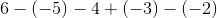Explanation:

To evaluate, simplify the plus and minus signs to a single sign.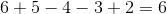### Example Question #21 : Concepts

Solve: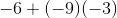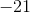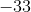Explanation:

Simplify the expression by eliminating the negative sign when multiplying two negative numbers.  Add the terms.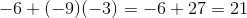### Example Question #22 : Concepts

Evaluate: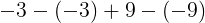Explanation:

In order to solve this expression, first simplify the double negative signs, which will convert to a positive sign.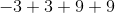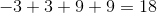The answer is:### Example Question #621 : Hspt Mathematics

Evaluate: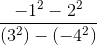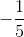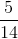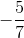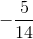Explanation:

Evaluate the numerator first by order of operations.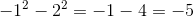Evaluate the denominator by order of operations.  Double negatives will equal a positive.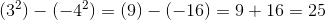Divide the numerator with the denominator and reduce the fraction.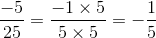### Example Question #22 : Concepts

Perform the indicated operation: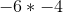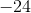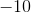Explanation:

When multiplying two negative numbers, their product becomes positive. Thus,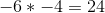### Example Question #22 : Concepts

When evaluating the expression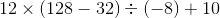,

which operation must be performed second?

Multiplication

Subtraction

Division

Multiplication

Explanation:

According to the order of operations, the operation inside the parentheses, which is the subtraction, is performed first.

This leaves a multiplication, a division, and an addition. By the order of operations, the multiplication and the division come next in left-to-right order; we choose the one that is to the left, which is the multiplication.

### Example Question #23 : Arithmetic

When evaluating the expression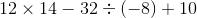,

which operation must be performed third?

Subtraction

Multiplication

Division